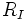# Codimension of an ideal

Let$R$ be a commutative unital ring and$I$ an ideal in$R$. The codimension or height of$I$ is defined as follows:
• If$I$ is a prime ideal, it is defined as the Krull dimension of the localization$R_I$
• Otherwise, it is defined to be the minimum of the Krull dimensions of prime ideals containing$I$